# 一文全理解 Python 中的面向对象编程？

XAMPP案例 792浏览

Python支持多种类型的编程范式，例如过程式编程、函数式编程、面向对象编程，而且还可以融合多种类型的范式。

• 创建Python类
• 数据属性
• 实例方法
• 属性
• 类和静态方法
• 继承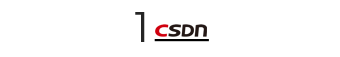``````>>> class MyClass:
...     pass
...
``````

``````>>> a = MyClass()
>>> a
<__main__.MyClass object at 0x7f32ef3deb70>``````

``````>>> type(a)
<class '__main__.MyClass'>
>>> a.__class__
<class '__main__.MyClass'>
>>> a.__class__.__name__
'MyClass'
``````

``````>>> type(MyClass)
<class 'type'>
``````

Python中每个实例方法的第一个参数必须对应于该实例，即该对象本身。按照惯例，这个参数名为self。后面是其他参数（如果有需要的话）。在调用方法时，我们无需明确提供与参数self相对应的参数。

``````>>> class MyClass:
...     def __init__(self, arg_1, arg_2, arg_3):
...         print(f'an instance of {type(self).__name__} created')
...         print(f'arg_1: {arg_1}, arg_2: {arg_2}, arg_3: {arg_3}')
...
``````

``````>>> a = MyClass(2, 4, 8)
an instance of MyClass created
arg_1: 2, arg_2: 4, arg_3: 8
``````

• 创建一个MyClass类型的对象的实例。
• 自动调用该实例的方法.__init__()。
• 我们传递给MyClass()方法的参数：（2，4和8）会被传递给.__init__()。
• .__init__()执行我们的请求，并输出结果。它利用type(self).__name__获取类的名称。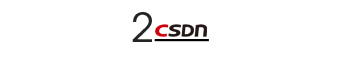``````>>> class MyClass:
...     def __init__(self, arg_1, arg_2, arg_3):
...         self.x = arg_1
...         self._y = arg_2
...         self.__z = arg_3
...
``````

• .x可以获取arg_1的值
• ._y可以获取arg_2的值
• .__ z可以获取arg_3的值

``````>>> class MyClass:
...     def __init__(self, arg_1, arg_2, arg_3):
...         self.x, self._y, self.__z = arg_1, arg_2, arg_3
...``````

• 开头没有下划线的属性（比如.x）通常可供对象外部的调用和修改。
• 开头拥有一个下划线的属性（比如._y）通常也可以从对象外部调用和修改。然而，下划线是一种惯用的标志，即该类的创建者强烈建议不要使用该变量。应该仅通过类的功能成员（比如方法和属性）调用和修改该变量。
• 开头拥有双下划线的属性（比如.__ z）将在名字修饰过程中被改名（在本例中它将被改名为._MyClass__z）。你也可以通过这个新名称从对象外部调用和修改它们。但是，我强烈反对这种做法。应该尽通过类的功能成员以其原始名称进行调用和修改。
Python对象的数据属性通常存储在名为.__ dict__的字典中，它也是对象的属性之一。但是，你也可以将数据属性存储在其他地方。我们可以直接访问__dict__，或利用Python的内置函数vars()获取.__ dict__：
``````>>> a = MyClass(2, 4, 8)
>>> vars(a)
{'x': 2, '_y': 4, '_MyClass__z': 8}
>>> a.__dict__
{'x': 2, '_y': 4, '_MyClass__z': 8}
``````

``````>>> a.x
2
>>> a._y
4
>>> a.__z
Traceback (most recent call last):
File "<stdin>", line 1, in <module>
AttributeError: 'MyClass' object has no attribute '__z'
>>> a.x = 16
>>> a.x
16
>>> vars(a)
{'x': 16, '_y': 4, '_MyClass__z': 8}``````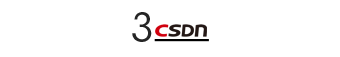●.set_z()：修改.__ z。
●.get_z()：返回.__ z的值。

``````>>> class MyClass:
...     def __init__(self, arg_1, arg_2, arg_3):
...         self.x, self._y, self.__z = arg_1, arg_2, arg_3
...
...     def set_z(self, value):
...         self.__z = value
...
...     def get_z(self):
...         return self.__z
...
>>> b = MyClass(2, 4, 8)
``````

``````>>> b.get_z()
8
>>> b.set_z(16)
>>> vars(b)
{'x': 2, '_y': 4, '_MyClass__z': 16}``````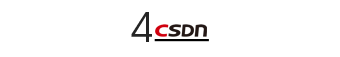``````>>> class MyClass:
...     def __init__(self, arg_1, arg_2, arg_3):
...         self.x, self._y, self.__z = arg_1, arg_2, arg_3
...
...     @property
...     def z(self):
...         return self.__z
...
...     @z.setter
...     def z(self, value):
...         self.__z = value
...
>>> b = MyClass(2, 4, 8)
``````

``````>>> b.z
8
>>> b.z = 16
>>> vars(b)
{'x': 2, '_y': 4, '_MyClass__z': 16}``````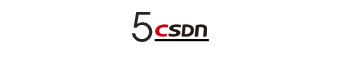``````>>> class MyClass:
...     def __init__(self, arg_1, arg_2, arg_3):
...         self.x, self._y, self.__z = arg_1, arg_2, arg_3
...
...     def f(self, arg):
...         print('instance method f called')
...         print(f'instance: {self}')
...         print(f'instance attributes:\n{vars(self)}')
...         print(f'class: {type(self)}')
...         print(f'arg: {arg}')
...
...     @classmethod
...     def g(cls, arg):
...         print('class method g called')
...         print(f'cls: {cls}')
...         print(f'arg: {arg}')
...
...     @staticmethod
...     def h(arg):
...         print('static method h called')
...         print(f'arg: {arg}')
...
>>> c = MyClass(2, 4, 8)``````

Python中常见的调用实例方法的方法如下：
``````>>> c.f('my-argument')
instance method f called
instance: <__main__.MyClass object at 0x7f32ef3def98>
instance attributes:
{'x': 2, '_y': 4, '_MyClass__z': 8}
class: <class '__main__.MyClass'>
arg: my-argument
``````

``````>>> MyClass.g('my-argument')
class method g called
cls: <class '__main__.MyClass'>
arg: my-argument
>>> MyClass.h('my-argument')
static method h called
arg: my-argument``````

``````>>> c.g('my-argument')
class method g called
cls: <class '__main__.MyClass'>
arg: my-argument
>>> c.h('my-argument')
static method h called
arg: my-argument
``````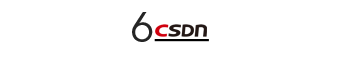``````>>> class MyOtherClass(MyClass):
...     def __init__(self, u, v, w, x, y, z):
...         super().__init__(x, y, z)
...         self.__u, self.__v, self.__w = u, v, w
...
...     def f_(self, arg):
...         print('instance method f_ called')
...         print(f'instance: {self}')
...         print(f'instance attributes:\n{vars(self)}')
...         print(f'class: {type(self)}')
...         print(f'arg: {arg}')
...
>>> d = MyOtherClass(1, 2, 4, 8, 16, 32)
``````

``````>>> vars(d)
{'x': 8,
'_y': 16,
'_MyClass__z': 32,
'_MyOtherClass__u': 1,
'_MyOtherClass__v': 2,
'_MyOtherClass__w': 4}
``````

``````>>> d.f('some-argument')
instance method f called
instance: <__main__.MyOtherClass object at 0x7f32ef3e7048>
instance attributes:
{'x': 8,
'_y': 16,
'_MyClass__z': 32,
'_MyOtherClass__u': 1,
'_MyOtherClass__v': 2,
'_MyOtherClass__w': 4}
class: <class '__main__.MyOtherClass'>
arg: some-argument
>>> d.f_('some-argument')
instance method f_ called
instance: <__main__.MyOtherClass object at 0x7f32ef3e7048>
instance attributes:
{'x': 8,
'_y': 16,
'_MyClass__z': 32,
'_MyOtherClass__u': 1,
'_MyOtherClass__v': 2,
'_MyOtherClass__w': 4}
class: <class '__main__.MyOtherClass'>
arg: some-argument``````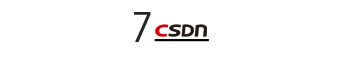• 方法：.__repr__()和.__str__()
• 方法：.__new__()
• 操作符
• 方法：.__getattribute__()、.__getattr__()、.__setattr__()和.__delattr__()
• 生成器
• 可调用性
• 创建序列
• 描述器
• 上下文管理
• 抽象类和成员
• 多重继承
• 使用super()
• 拷贝
• 序列化
• slot
• 类修饰器
• 数据类